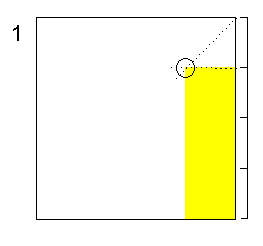Origami Heaven

The website of writer and paperfolding designer David Mitchell

x

 The Twin Platinum Rectangles and the Mock Platinum Rectangle

The twin platinum rectangles are significant in modular origami design since their natural folding geometry yields angles of 108, 72 and 36 degrees.

The platinum rectangles are defined as those which contain the twin golden proportion triangles arranged apex to apex.The thin platinum rectangle.The fat platinum rectangle. In both diagrams x:y is the golden proportion.

The thin platinum rectangle is very close to a 3:1 rectangle and this simple approximation has long been in use by origami designers.

The diagrams below show how a mock (fat) platinum rectangle can be approximated from a square (or indeed any other rectangle). It has the advantage that those location creases which are not significant in terms of its natural folding geometry lie almost entirely outside the area of the final rectangle.1, Find the top left-hand corner of an imaginary 3 by 1 rectangle lying within the square by creasing in part of one diagonal and part of a three-quarter way line. The point where they intersect is the top left corner of the rectangle. 2. Use the corner of this imaginary 3 x 1 rectangle as a location point for this fold. When the fold is flattened the crease must pass exactly through corner z.3, Use the point where the new crease intersects the left hand raw edge to locate the final fold. 4, Cut along the new crease to separate the mock platinum rectangle from the square.